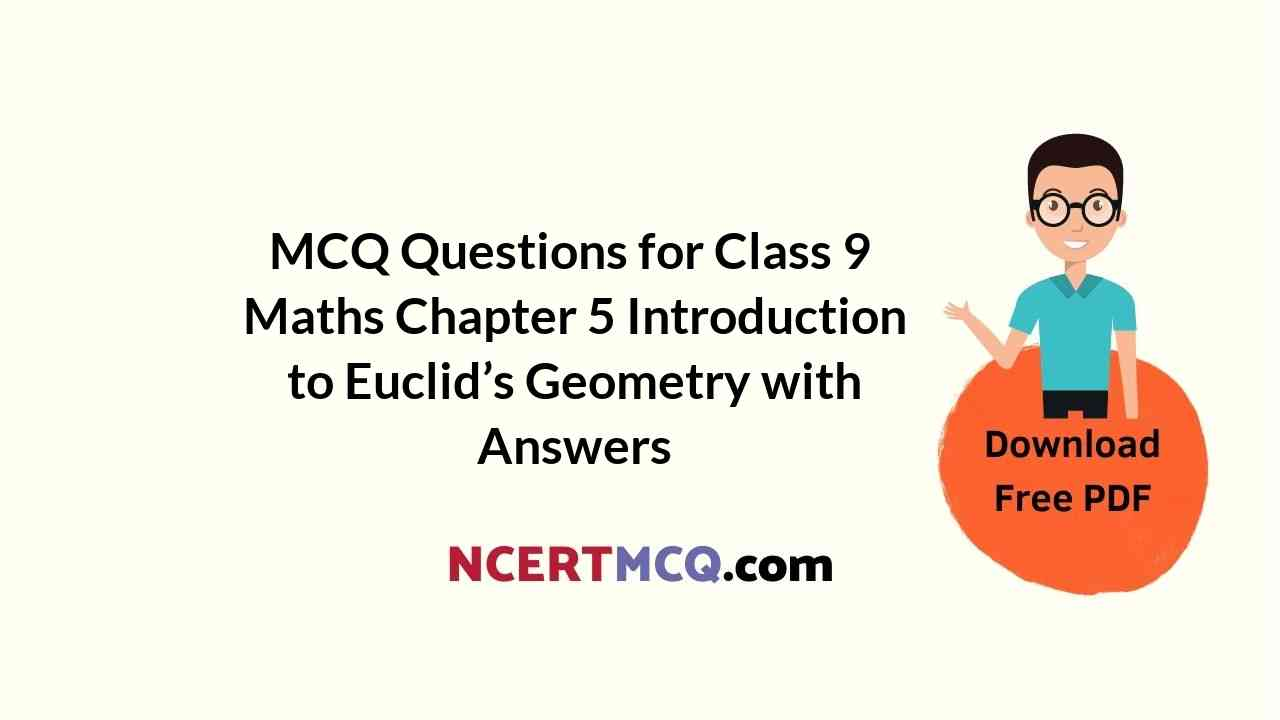Check the below NCERT MCQ Questions for Class 9 Maths Chapter 5 Introduction to Euclid’s Geometry with Answers Pdf free download. MCQ Questions for Class 9 Maths with Answers were prepared based on the latest exam pattern. We have provided Introduction to Euclid’s Geometry Class 10 Maths MCQs Questions with Answers to help students understand the concept very well. https://ncertmcq.com/mcq-questions-for-class-9-maths-with-answers/

Students can also refer to NCERT Solutions for Class 9 Maths Chapter 5 Introduction to Euclid’s Geometry for better exam preparation and score more marks.

## Introduction to Euclid’s Geometry Class 9 MCQs Questions with Answers

Class 9 Maths Chapter 5 MCQ Question 1.
The number of dimensions, a solid has:
(a) 1
(b) 2
(c) 3
(d) 0

MCQ Questions For Class 9 Maths Ncert Chapter 5 Question 2.
The total number of propositions in the elements are:
(a) 465
(b) 460
(c) 13
(d) 55

Class 9 Maths Chapter 5 MCQ With Answers Question 3.
In Indus valley civilisation (about 3000 BC), the bricks used for construction work were having dimensions in the ratio
(a) 1 : 3 : 4
(b) 4 : 2 : 1
(c) 4 : 4 : 1
(d) 4 : 3 : 2

Answer: (b) 4 : 2 : 1

Euclid Geometry Class 9 MCQ Question 4.
The things which are double of same thing are
(a) equal
(b) halves of same thing
(c) unequal
(d) double of the same thing

Introduction To Euclids Geometry Class 9 MCQ Question 5.
Which of the following statements is incorrect?
(а) A line segment has definite length.
(b) Three lines are concurrent if and only if they have a common point.
(c) Two lines drawn in a plane always intersect at a point.
(d) One and only one line can be drawn passing through a given point and parallel to a given line.

Answer: (c) Two lines drawn in a plane always intersect at a point.

Chapter 5 Maths Class 9 MCQ Question 6.
Select the wrong statement:
(а) Only one line can pass through a single point.
(b) Only one line can pass through two distinct points.
(c) A terminated line can be produced indefinitely on both the sides.
(d) If two circles are equal, then their radii are equal.

Answer: (а) Only one line can pass through a single point.

Introduction To Euclid’s Geometry Class 9 MCQ Question 7.
Which one of the following statements is true?
(a) Only one line can pass through a single point.
(b) There are an infinite number lines which pass through two distinct points.
(c) Two distinct lines cannot have more than one point in common.
(d) If two circles are equal, then their radii are not equal.

Answer: (c) Two distinct lines cannot have more than one point in common.

Ch 5 Maths Class 9 MCQ Question 8.
If the point P lies in between M and N and C is mid point of MP, then:
(a) MC + PN = MN
(b) MP + CP – MN
(c) MC + CN = MN
(d) CP + CN = MN

Answer: (c) MC + CN = MN

Class 9 Maths Ch 5 MCQ Question 9.
‘Lines are parallel if they do not intersect’ is stated in the form of:
(a) an axiom
(b) a definition
(c) a postulate
(d) a proof

Maths Class 9 Chapter 5 MCQ Question 10.
Two planes intersect each other to form a:
(a) plane
(b) point
(c) straight line
(d) angle

Class 9 Math Chapter 5 MCQ Question 11.
The number of lines that can pass through a given point is
(a) two
(b) none
(c) only one
(d) infinitely many

MCQ Questions For Class 9 Maths Chapter 5 Question 12.
How many lines do pass through two distinct points?
(a) 1
(b) 2
(c) 3
(d) 4

Class 9 Maths Chapter 5 MCQ Online Test Question 13.
The number of line segments determined by three collinear points is:
(a) two
(b) three
(c) only one
(d) four

Euclids Geometry Class 9 MCQ Question 14.
Number of dimension(s) a surface has:
(a) 0
(b) 1
(c) 2
(d) 3

Class 9 Chapter 5 Maths MCQ Question 15.
Euclid stated that things which are equal to the same thing are equal to one another in the form of:
(a) an axiom
(b) a definition
(c) a postulate
(d) a proof

Question 16.
The things which coincide with one another are:
(a) equal
(b) unequal
(c) half of same thing
(d) triple of one another

Question 17.
Given four points such that no three of them are collinear, then the number of lines that can be drawn through them is:
(a) 2 lines
(b) 4 lines
(c) 6 lines
(d) 8 lines

Question 18.
Two intersecting lines cannot be parallel to the same line, is stated in the form of:
(a) an axiom
(b) a definition
(c) a postulate
(d) a proof

Question 19.
Euclid stated that all right angles are equal to each other in the form of:
(a) an axiom
(b) a definition
(c) a postulate
(d) a proof

Question 20.
Which of the following needs a proof?
(a) theorem
(b) Axiom
(c) Definition
(d) Postulate